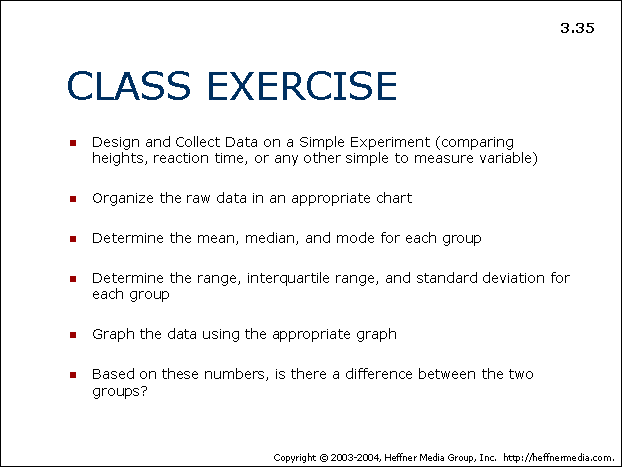# 35: Class Exercise: Simple Experiment## CLASS EXERCISE

• Design and Collect Data on a Simple Experiment (comparing heights, reaction time, or any other simple to measure variable)
• Organize the raw data in an appropriate chart
• Determine the mean, median, and mode for each group
• Determine the range, interquartile range, and standard deviation for each group
• Graph the data using the appropriate graph
• Based on these numbers, is there a difference between the two groups?